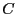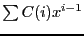Next: Linear algebra Up: Parametric polynomials and eigenvalues Previous: Implementation   Contents

Coefficient of the characteristic polynomial

In some cases it may be difficult to obtain the analytical form of the coefficients of the characteristic polynomial of a matrix. The following procedure allows to compute these coefficients even if the matrix is an interval matrix:

INTERVAL_VECTOR Coeff_CharPoly(int Size,INTERVAL_MATRIX &A)
where Size is the dimension of the matrix and A the matrix. This procedures returns the coefficients in an interval vectorand the polynomial is.

Jean-Pierre Merlet 2012-12-20